# How to VLOOKUP multiple values in Excel based on one or several criteria

The tutorial shows a few ways to Vlookup multiple matches in Excel based on one or more conditions and return multiple results in a column, row or single cell.

When using Microsoft Excel for data analysis, you may often find yourself in situations when you need to get all matching values for a specific id, name, email address or some other unique identifier. An immediate solution that comes to mind is using the Excel VLOOKUP function, but the problem is that it can only return a single match.

Vlookup for multiple values can be done via a combined use of several functions. If you are far from being an Excel expert, don't hurry to leave this page. I will do my best to explain the underlying logic so that even a novice could understand the formulas and adjust them for solving similar tasks. Even better, I will show you another possible solution that takes just a few mouse clicks and does not require any knowledge of Excel formulas at all!

## How to do multiple Vlookup in Excel using a formula

As mentioned in the beginning of this tutorial, there is no way to make Excel VLOOKUP return multiple values. The task can be accomplished by using the following functions in an array formula:

• IF - evaluates the condition and returns one value if the condition is met, and another value if the condition is not met.
• SMALL - gets the k-th smallest value in the array.
• INDEX - returns an array element based on the row and column numbers you specify.
• ROW - returns the row number.
• COLUMN - returns the column number.
• IFERROR - traps errors.

Below you will find a few examples of such formulas.

### Formula 1. Vlookup multiple matches and return results in a column

Let's say, you have the seller names in column A and the products they sold in column B, column A containing a few occurrences of each seller.  Your goal is to get a list of all products sold by a given person. To have it done, please follow these steps:

1. Enter a list of unique names in some empty row, in the same or another worksheet. In this example, the names are input in cells D2:G2: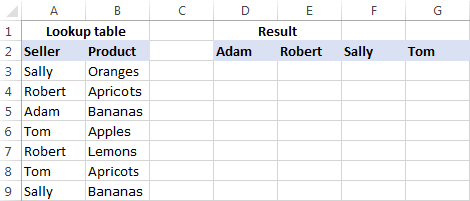Tip. To quickly get all different names in a list, you can use a formula to extract distinct values in Excel.
2. Under the first name, select a number of empty cells that is equal to or greater than the maximum number of possible matches, enter one of the following array formulas in the formula bar, and press Ctrl + Shift + Enter to complete it (in this case, you will be able to edit the formula only in the entire range where it's entered). Or, you can enter the formula in the first cell, hit Ctrl + Shift + Enter, and then copy the formula to a few more cells down the column (in this case, you will be able to edit the formula in each cell individually).

`=IFERROR(INDEX(\$B\$3:\$B\$13, SMALL(IF(D\$2=\$A\$3:\$A\$13, ROW(\$B\$3:\$B\$13)-2,""), ROW()-2)),"")`

or

`=IFERROR(INDEX(\$B\$3:\$B\$13,SMALL(IF(D\$2=\$A\$3:\$A\$13,ROW(\$A\$3:\$A\$13)- MIN(ROW(\$A\$3:\$A\$13))+1,""), ROW()-2)),"")`

As you see, the 1st formula is a bit more compact, but the 2nd one is more universal and requires fewer modifications (we will elaborate more on the syntax and logic a bit further).

3. Copy the formula to other columns. For this, select the range of cells where you've just entered the formula, and drag the fill handle (a small square at the lower right-hand corner of the selected range) to the right.

The result will look something similar to this: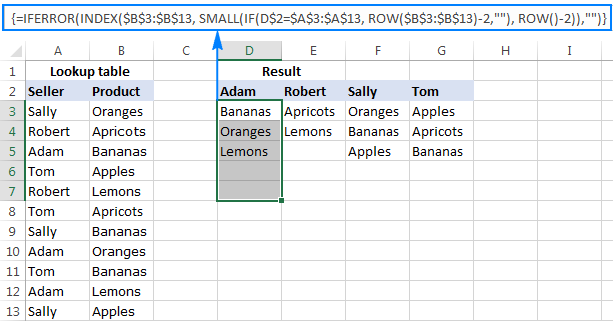#### How this formula works

This is an example of intermediate to advanced uses of Excel that implies basic knowledge of array formulas and Excel functions. Working from the inside out, here's what you do:

1. IF function

At the core of the formula, you use the IF function to get the positions of all occurrences of the lookup value in the lookup range: IF(D\$2=\$A\$3:\$A\$13, ROW(\$B\$3:\$B\$13)-2,"")

IF compares the lookup value (D2) with each value in the lookup range (A3:A13), and if the match if found, returns the relative position of the row; an empty string ("") otherwise.

The relative positions of the rows are calculated by subtracting 2 from ROW(\$B\$3:\$B\$13) so that the first row has position 1. If your return range begins in row 2, then subtract 1, and so on. The result of this operation is the array {1;2;3;4;5;6;7;8;9;10;11}, which goes to the value_if_true argument of the IF function.

Instead of the above calculation, you can use this expression: ROW(lookup_column)- MIN(ROW(lookup_column))+1, which returns the same result but does not require any changes regardless of the return column location. In this example, it'd be ROW(\$A\$3:\$A\$13)- MIN(ROW(\$A\$3:\$A\$13))+1.

So, at this point you have an array consisting of numbers (positions of matches) and empty strings (non-matches). For cell D3 in this example, we have the following array: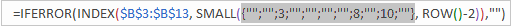If you check with the source data, you will see that "Adam" (lookup value in D2) appears on the 3rd, 8th and 10th positions in the lookup range (A3:A13).

2. SMALL function
Next, the SMALL(array, k) function steps in to determine which of the matches should be returned in a specific cell.

With array already established, let's work out the k argument, i.e. the k-th smallest value to be returned. For this, you make a sort of an "incremental counter" ROW()-n, where "n" is the row number of the first formula cell minus 1. In this example, we entered the formula in cells D3:D7, so ROW()-2 returns "1" for cell D3 (row 3 minus 2), "2" for cell D4 (row 4 minus 2), etc.

As the result, the SMALL function pulls the 1st smallest element of the array in cell D3, the 2nd smallest element in cell D4, and so on. And this transforms the initial long and complex formula into a very simple one, like this: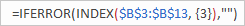Tip. To see the calculated value behind a certain part of the formula, select that part in the formula bar and press F9.
3. INDEX function

This part is easy. You use the INDEX function to return the value of an array element based on its row number.

4. IFERROR function

And finally, you wrap the formula in the IFERROR function to handle possible errors, which are inevitable because you cannot know how many matches will be returned for this or that lookup value, and therefore you copy the formula to a number of cells equal to or greater than the number of possible matches. Not to scare your users with a bundle of errors, simply replace them with an empty string (blank cell).

Note. Please notice the proper use of absolute and relative cell references in the formula. All references are fixed except for the relative column reference in the lookup value (D\$2), which should change based on a relative position of a column(s) where the formula is copied to return matches for other lookup values.

Putting all this together, we get the following generic formulas to Vlookup multiple values in Excel:

Formula 1:

IFERROR(INDEX(return_range, SMALL(IF(lookup_value = lookup_range, ROW(return_range )- m ,""), ROW() - n )),"")

Formula 2:

IFERROR(INDEX(return_range, SMALL(IF(lookup_value = lookup_range , ROW(lookup_range) - MIN(ROW(lookup_range ))+1,""), ROW() - n)),"")

Where:

• m is the row number of the first cell in the return range minus 1.
• n is the row number of the first formula cell minus 1.
Note. In the above example, both n and m are equal to "2" because our return range and formula range both begin in row 3. In your worksheets, these may be different numbers.

### Formula 2. Vlookup multiple matches and return results in a row

In case you want to return multiple values in rows rather than columns, change the above formulas this way:

`=IFERROR(INDEX(\$B\$3:\$B\$13, SMALL(IF(\$D3=\$A\$3:\$A\$13, ROW(\$B\$3:\$B\$13)-2,""), COLUMN()-4)),"")`

Or

`=IFERROR(INDEX(\$B\$3:\$B\$13,SMALL(IF(\$D3=\$A\$3:\$A\$13,ROW(\$A\$3:\$A\$13)- MIN(ROW(\$A\$3:\$A\$13))+1,""),COLUMN()-4)), "")`

Like in the previous example, both are array formulas, so remember to press the Ctrl + Shift + Enter shortcut to complete them correctly.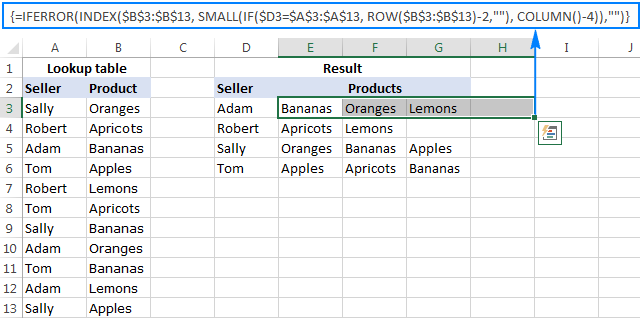The formulas work with the same logic as in the previous example, except that you use the COLUM function instead of ROW to determine which matching value should be returned in a specific cell: COLUMN()-n. Where n is the column number of the first cell where the formula is entered minus 1. In this example, the formula is input in cells E2:H2. With E being the 5th column, n is equal to "4" (5-1=4).

Note. For the formula to get copied correctly to other rows, mind the lookup value references, absolute column and relative row, like \$D3.

Wrapping up, here are the generic formulas for Vlookup with multiple results returned in rows:

Formula 1:

IFERROR(INDEX(return_range, SMALL(IF(lookup_value = lookup_range, ROW(return_range) - m, ""), COLUMN() - n)), "")

Formula 2:

IFERROR(INDEX(return_range, SMALL(IF(lookup_value = lookup_range, ROW(lookup_range) - MIN(ROW( lookup_range))+1,""),COLUMN() - n)), "")

Where:

• m is the row number of the first cell in the return range minus 1.
• n is the column number of the first formula cell minus 1.

### Formula 3. Vlookup multiple matches based on multiple conditions

You already know how to Vlookup for multiple values in Excel based on one condition. But what if you want to return multiple matches based on two or more criteria? Taking the previous examples further, what if you have an additional Month column, and you are looking to get a list of all products sold by a given seller in a specific month?

If you are familiar with arrays formulas, you may remember that they allow using asterisk (*) as the AND operator. So, you can just take the formulas discussed in the two previous examples, and have them check multiple conditions as demonstrated below.

#### Return multiple matches in a column

IFERROR(INDEX(return_range, SMALL(IF(1=((--(lookup_value1=lookup_range1)) * ( --(lookup_value2=lookup_range2))), ROW(return_range)-m,""), ROW()-n)),"")

Where:

• m is the row number of the first cell in the return range minus 1.
• n is the row number of the first formula cell minus 1.

Assuming the Seller list (lookup_range1) is in A3:A30, the Month list (lookup_range2) is in B3:B30, the seller of interest (lookup_value1) is in cell E3, and the month of interest (lookup_value2) is in cell F3, the formula takes the following shape:

`=IFERROR(INDEX(\$C\$3:\$C\$30, SMALL(IF(1=((--(\$E\$3=\$A\$3:\$A\$30)) * (--(\$F\$3=\$B\$3:\$B\$30))), ROW(\$C\$3:\$C\$30)-2,""), ROW()-2)),"")`

This layout may be useful for creating a dashboard, e.g. your users can enter a name in E3, month in F3 and get a list of products in column G: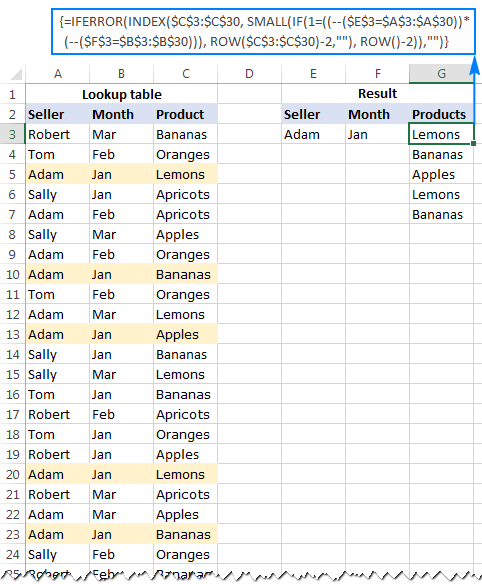#### Return multiple results in a row

If you want to pull multiple values based on multiple criteria sets, you may prefer the horizontal layout where results are returned in rows. In this case, use this following generic formula:

IFERROR(INDEX(return_range, SMALL(IF(1 = ((--(lookup_value1=lookup_range1)) * (--(lookup_value2 = lookup_range2))), ROW(return_range) - m, ""), COLUMN() - n)),"")

Where:

• m is the row number of the first cell in the return range minus 1.
• n is the row number of the first formula cell minus 1.

For our sample dataset, the formula goes as follows:

`=IFERROR(INDEX(\$C\$3:\$C\$30, SMALL(IF(1=((--(\$E3=\$A\$3:\$A\$30)) * (--(\$F3=\$B\$3:\$B\$30))), ROW(\$C\$3:\$C\$30)-2,""), COLUMN()-6)),"")`

And the result can resemble this: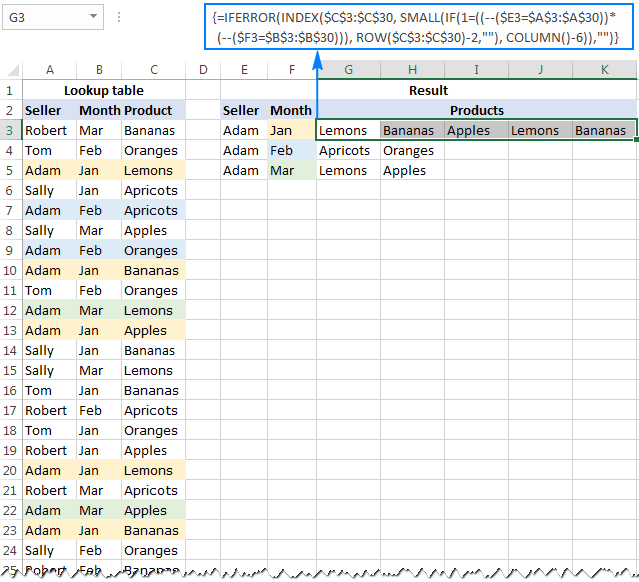In a similar manner, you can do multiple Vlookup with three, four or more conditions.

#### How these formulas work

Basically, the formulas to Vlookup multiple values with multiple conditions work with the already familiar logic, explained in the very first example. The only difference is that the IF function now tests multiple conditions:

1=((--(lookup_value1=lookup_range1))*(--(lookup_value2=lookup_range2))*…)

The result of each lookup_value=lookup_range comparison is an array of logical values TRUE (condition is met) and FALSE (condition is not met). The double unary operator (--) coerces the logical values into 1's and 0's. And because multiplying by zero always gives zero, in the resulting array, you have 1 only for those elements that meet all of the specified conditions. Now, you simply compare the final array with number 1 so that the ROW function returns the numbers of rows that meet all the conditions, an empty string otherwise.

A word of caution. All of the multiple Vlookup formulas discussed in this tutorial are array formulas. As such, each formula iterates through all elements of the arrays every time the source data is changed or the worksheet is recalculated. On large worksheets containing hundreds or thousands of rows, this may significantly slow down your Excel.

This is how you Vlookup multiple matches in Excel using formulas. To have a closer look at the examples and probably reverse-engineer the formulas for better understanding, you are welcome to download the Excel Vlookup Multiple Values sample worksheet.

## How to Vlookup to return multiple values in one cell

I will be upfront - I don't know a formula to Vlookup duplicates that would output multiple matches in a single sell. However, I do know a formula-free (read "stress-free" :) way to do this, by using two add-ins included with our Ultimate Suite for Excel. If you don't have it in your Excel yet, you can download a free 14-day trial version here, and then follow the steps outlined below.

#### Source data and expected result

As shown in the screenshot, we continue working with the dataset we've used in the previous example. But this time we want to achieve something different - instead of extracting multiple matches in separate cells, we want them to appear in a single sell, separated with a comma, space, or some other delimiter of your choosing.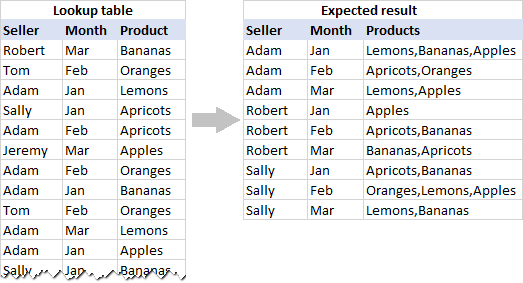#### Pull rows with multiple matches to the main table

In your main table, enter a list of unique names in the first column, months in the second column, and arrange them like shown in the screenshot below. After that, carry out the following steps:

1. Select your main table or click any cell within it, and then click the Merge Two Tables button on the ribbon: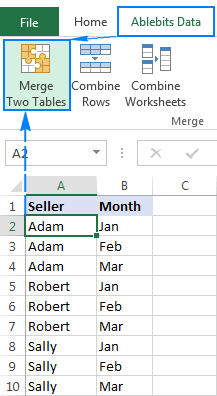2. The add-in is smart enough to identify and pick the entire table, so you just click Next: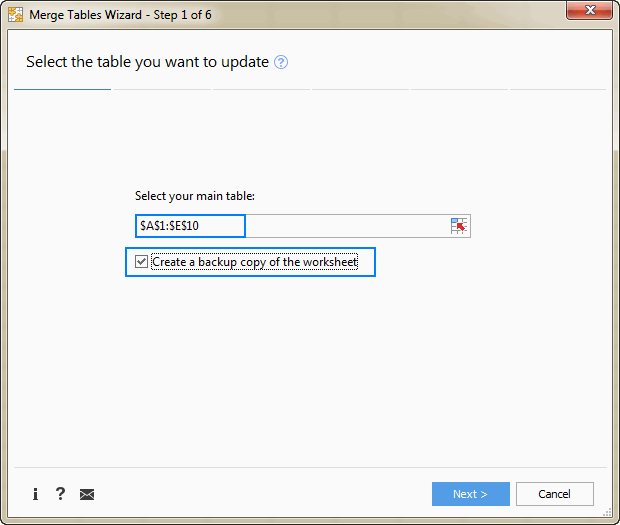Tip. When using the tool for the first time, it stands to reason to select the Create a backup copy of the worksheet box in case something goes wrong.
3. Select the lookup table, and click Next.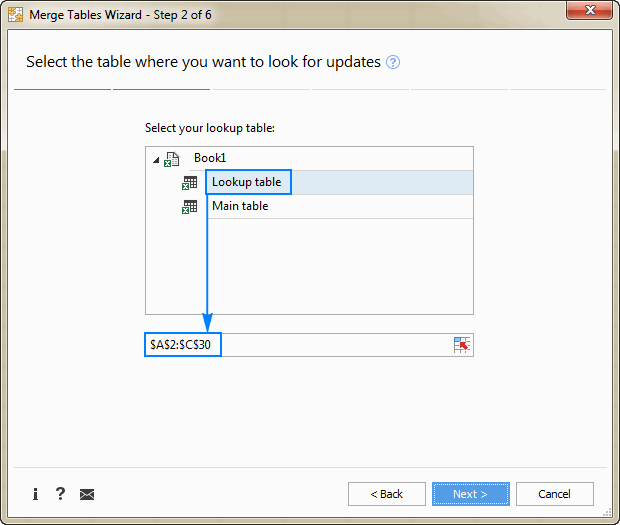4. Choose one or more matching pairs of columns that should be compared in the main table and lookup table (in this example, it's the Seller and Month columns), and then click Next.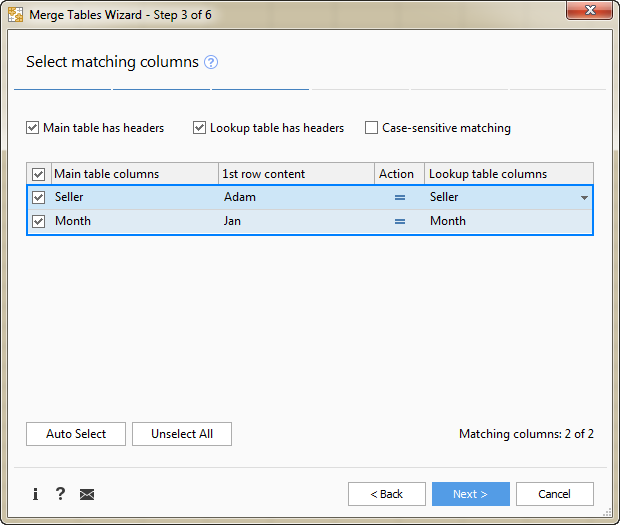5. Select the column(s) from which you want to pull matching values (Product in this example), and click Next.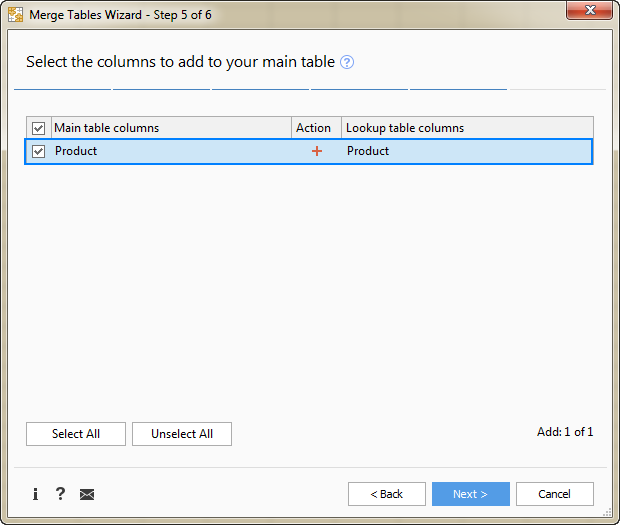6. Tell the add-in how exactly you want multiple matches to be arranged in the main table. For this example, we need the following option: Insert rows with duplicate matching values after the row with the same value. Make sure that no other option is selected and click Finish.At this point, you will have the following result - all matching rows are pulled to the main table and grouped by the values in the lookup columns - first by Seller, and then by Month: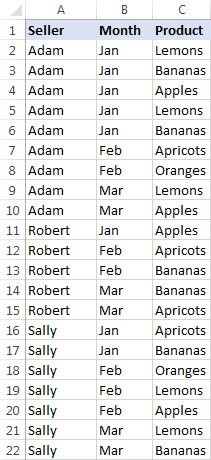The resulting table already looks nice, but it's not exactly what we wanted, right? As you remember, we are looking to Vlookup multiple matches and have them returned in a single sell, comma or otherwise separated.

#### Combine duplicates rows into one row

To merge "duplicate rows" in a single row, we are going to use another tool - Combine Rows Wizard.

1. Select the table produced by the Merge Tables tool (please see the screenshot above) or any cell within the table, and click the Combine Rows button on the ribbon: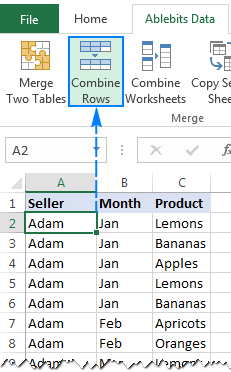2. Check if the add-in's got the table right, and click Next: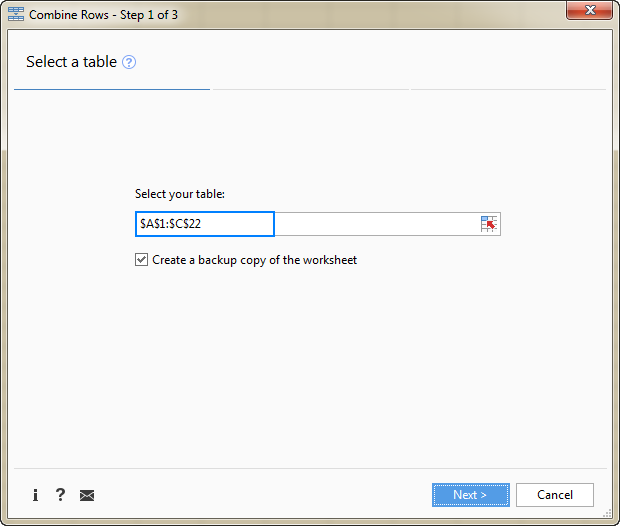3. Select the key column or columns (Seller and Month in this example), and click Next: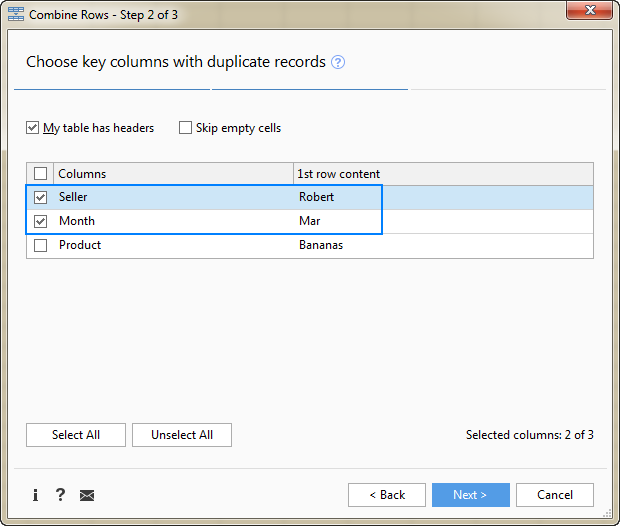4. Select the column(s) that contains multiple matches (Product in this example), choose the desired delimiter (semicolon, comma, space or line break), and click Finish.

Optionally, you can enable one of the additional features, or both:

• Delete duplicate values - if the column to be merged contains a few identical values, the first occurrence will be kept, duplicate matches will be deleted.

In this example, we do not check this option, and the add-in returns all found matches. For example, in cell C2, we have this string: Lemons, Bananas, Apples, Lemons, Bananas (please see the result on step 5 below). If you choose to delete duplicates, the result would be: Lemons, Bananas, Apples.

• Skip empty cells - self-explanatory :)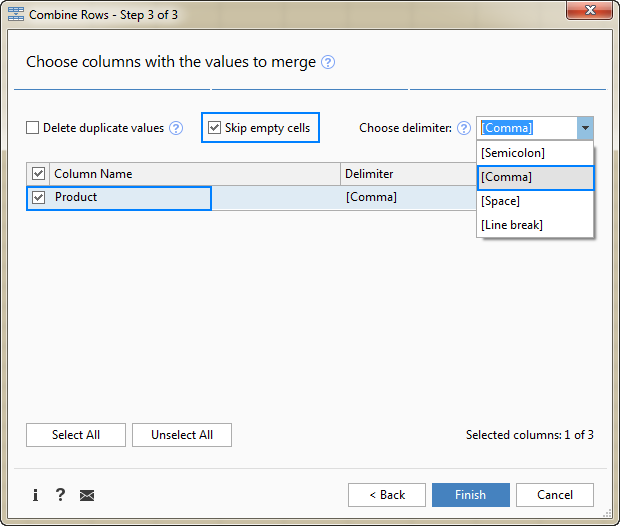5. Allow the add-in a few seconds for processing, and you are all set!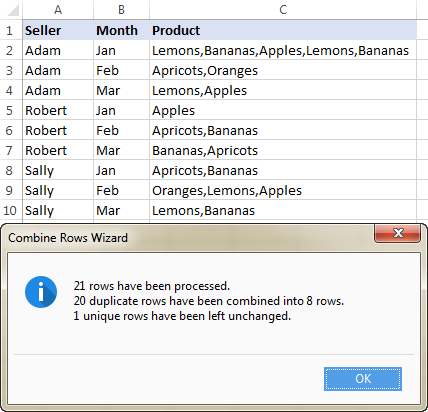This is how you can look up and return multiple values in Excel using our tools. If you are curious to give them a try, a fully-functional evaluation version of the Ultimate Suite can be downloaded here. If the add-ins prove useful in your work, be sure to grab the 15% off coupon code that we provide exclusively to our blog readers: AB14-BlogSpo.

I thank you for reading and hope to see you on our blog next week!

### 192 Responses to "How to VLOOKUP multiple values in Excel based on one or several criteria"

1.R Banks says:

Good day !
Using the row array formula =IFERROR(INDEX(\$B\$3:\$B\$13,SMALL(IF(\$D3=\$A\$3:\$A\$13,ROW(\$A\$3:\$A\$13)- MIN(ROW(\$A\$3:\$A\$13))+1,""),COLUMN()-4)), ""), let's say that Adam's first line column B is blank rather than "Bananas" - how can I skip the blank and show the next real value in cell E1? (in other words, E1 would be "Oranges")
Thanks!

•Dustin says:

Did you find the answer to this question?

2.jason says:

Hi

I need some help to do retrieve information from an excel sheet and populate specific columns in another excel sheet within the same workbook. I tried this but I have given up with the formula to get it working.

For examle,
1. Sheet 1 is the master sheet where I enter the information into each cell.
For example,
Device ID Device MAC Hostname Service Tag
FS-001 Z9264F-ON 20040F0E3B4E frxsw-ds01 H5WMXC2

3.jason says:

I need help to make a formula to retrieve information from a 'master' sheet (where information is entered manually) and populate specific cells in a 'target' sheet to avoid re-entering this information.

For example,

Master sheet
-----------

A B C D
1 Device ID Device MAC Hostname Service Tag
2 FS-001 00:00:00:00:00:00 device01 12345

Target Sheet
----------

A B C D E
1 Device ID Hostname IP address Device MAC Gateway
2 FS-001 device01 00:00:00:00:00:00

So, if on the 'Target' sheet A2 = 'Master' sheet A2's value of FS-001, then values from Master sheet B2 and C2 will be populated
in the cells of 'Target' sheet D2 and B2 respectively.

•Amy says:

Have you found the formula for this? I need a similar one too.

4.Salim says:

I need help to get Max value from set value but if any data is 0 ( zero) then return result should be 0(zero). Pls see below data & result what I want. Pls advise.
A 20
B 20
C 30
A 40
B 10
D 60
B 35
D 40
A 0
D 0
A 40

Result should be
A 0
B 35
C 30
D 0

•Scott says:

This should do it. Assuming your data begins in cell A1. Make sure you hit Ctrl+Shift+Enter when finished as this is an array formula. {=IF(MIN(IF("A"=\$A\$1:\$A\$11,\$B\$1:\$B\$11,""))=0,0,MAX(IF("A"=\$A\$1:\$A\$11,\$B\$1:\$B\$11,"")))}

5.GOWHAR ALI says:

=VLOOKUP(E5,A1:B8,2,0)&", "&INDEX(B1:B8,MATCH(E5,A1:A8,0)+2)

6.JYD says:

Dear Svetlana
Your pages on Excel are really incredible! I learned a lot, but understand that there is still so much to learn!

I am searching for a solution to retrieve data from an input sheet. When one selects one or more search terms in another sheet (from a pre-defined list) I would like to see all the results that meet the conditions. It is very similar to your examples here, "Vlookup multiple matches based on multiple conditions". But instead of AND I would need OR. Eg: if the search term is 'apples', I would like to see Excel giving me a list of all the apple sales. If the search terms are 'apples' and 'bananas', I would like to see Excel giving me a list of all the sales of apples and bananas. I am searching for hours ans hours, but cannot manage it... Maybe you have the solution?

7.syeda says:

Hi

I need some help with retrieving value from a table:
min max 1 2 3 4 5 6 7 8 9 10
0 8 0.00% 0.00% 2.50% 4.00% 5.00% 6.00% 7.00% 8.00% 9.00% 10.00%
9 10 5.00% 5.00% 7.50% 9% 10% 11% 12% 13% 14% 15%
11 24 7.50% 7.50% 10% 11.50% 12.50% 13.50% 14.50% 15.50% 16.50% 17.50%
25 49 10.00% 10.00% 12.50% 14% 15% 16% 17% 18% 19% 20%
50 99 12.50% 12.50% 15% 16.50% 17.50% 18.50% 19.50% 20.50% 21.50% 22.50%
100 199 15.00% 15.00% 17.50% 19% 20% 21% 22% 23% 24% 25%
200 >200 17.50% 17.50% 20% 21.50% 22.50% 23.50% 24.50% 25.50% 26.50% 27.50%

I am using the formula: =INDEX(\$E\$7:\$N\$13,MATCH(C3,D7:D13,2), MATCH(C10,Sheet1!E6:N6,0))
However, everytime it is reading only the first line

Can you help me out. Thanks for reading.

8.Tonya says:

Your information was very helpful, thank you!!! I want to use the formulas to do an "or" statement. I am using the formula {=IFERROR(INDEX('CTE Detail'!\$F\$2:\$F\$900000,SMALL(IF('CTE Detail'!\$M\$2:\$M\$900000=auto!\$F\$1,ROW('CTE Detail'!\$F\$2:\$F\$900000)-1,""),ROW()-8)),"")}. This works as long as each program only has one subject code. I am bringing 2 spreadsheets together. What I am looking for is all of the students in certain classes by subject code (auto!\$F\$1). I have some programs that have multiple subject codes, how do I write the formula? I believe the * is for an "and" statement. Thank you,

9.Michelle McNulty says:

I need to bring data from a master sheet where there is more than one entry, but might have slightly different data in a number of columns.

10.Bijay Shrestha says:

i need help
Rujan Shakya Absent
Abhishekh Wosti Present
Kshitiz Pokhrel Present
Rujan Shakya Present
in above details need to just take present name to another sheet

11.Larry says:

Hello - I tried to implement Formula 1 in this article, Vlookup multiple values and return results in a column, using the same data entered in the same cells in an Excel 2019 spreadsheet. But the formula doesn't work. To see why, I stripped out the IFERROR code and just ran the INDEX function without error checking. This showed that the formula produces a #Value error in the result. Does anyone know why the example is not working for me? I'm truly stumped.

•Svetlana Cheusheva (Ablebits.com Team) says:

Hi Larry,
It's difficult to say what the problem might be without seeing your data and formula. IFERROR is a very simple function and usually it does not cause any issues.

You can download our sample workbook with working formulas and copy/past the desired formula from there to try it on your data.

•James says:

I have a similar issue, happening with your sample workbook too. I open the sample workbook, all the formulas show exactly as on this site. If I then click in the formula bar and hit enter the calculated values that were there when I open the book disappear. The formula is still there it just returns the error result value. The only difference in the formula bar is that the {} brackets round the formula are no longer there after calculating the formula locally.

•Dave Williams says:

James,
You most likely need to re-apply the "Ctrl-Shift-Enter' command to let the formula know it is based on an ARRAY.
Put your cursor in the cell with the formula and press Control-Shift and Enter.
Just a thought...

12.Matt says:

This was so incredibly helpful! I looked at other tutorials prior to this, and none of them gave the background on "why" or "what" each step did so I couldn't manipulate the other examples to fit my needs. This was the only truly comprehensive guide I came across. Thank you so much!

13.DIEGO says:

14.AJ says:

doing similar to Formula 1. Vlookup multiple values and return results in a column

However I have the data in a separate sheet called 'Eg Data' hence I changed my formula to read as follows:
=IFERROR(INDEX('Eg Data'!\$B\$3:\$B\$13,SMALL(IF(D\$5='Eg Data'!\$A\$3:\$A\$13,ROW('Eg Data'!\$A\$3:\$A\$13)- MIN(ROW('Eg Data'!\$A\$3:\$A\$13))+1,""), ROW()-2)),"")
In example data all is the same as per example above in 'Formula 1. Vlookup multiple values and return results in a column' and using same cells (a3:b13)

the other only difference is that the name in my case 'Adam' is not in cell D2 as per example above but in D5 and I want the results in D6 no in D3 as per example above.

This seems to give a problem when I moved the name from D2 to D5 and results to be in D6 instead of D3

Does the formula need changing?

15.Francisco says:

This was a great tutorial, thank you very much! I got the formulas to work for my database, however I have an additional restriction. Bringing it to this example (I'm doing results in a ROW), let's say the products are listed as ORANGES - APRICOTS - BANANAS - APPLES - LEMONS. So if Adam sold Bananas, Oranges and Lemons, I want his results to show ORANGES - *blank* - BANANAS - *blank* - LEMONS. To make it easier to understand, my categories are time, so I need the results chronologically (6-10-12-14 hours). So if a result appears in 12 and 14, I want to see the 4 columns as blank-blank-result-result, but what I'm getting is result-result-blank-blank. Any thoughts?

16.Jay Dattani says:

Sally Oranges
Robert Apricots
Tom Apples
Robert Lemons
Tom Apricots
Sally Bananas
Tom Bananas
Sally Apples
X y

Please suggest what should I do if my range is extended till x & y. Simply changing range in your formula is not working.

17.TAHIRULHAQ says:

Please give me the result column c, as completed or incompleted when lookup from column a into column b.
Column (a) Column (b) Result Column (c)
OTE3‐L1‐IM‐300992‐01 12-Apr Completed
OTE3‐L1‐IM‐300992‐01 13-Apr Completed
OTE3‐L1‐IM‐300992‐02 13-Apr Completed
OTE3‐L1‐IM‐300992‐02 Incompleted
OTE3‐L1‐IM‐300992‐03 Incompleted
OTE3‐L1‐IM‐300992‐03 Incompleted
OTE3‐L1‐IM‐300992‐03 Incompleted
OTE3‐L1‐IM‐300992‐03 Incompleted

18.Bala says:

Thanks a lot for your effort you saved my time.

19.Nick says:

20.Ali says:

HI
When i use this code the excel have a error
=IFERROR(INDEX(\$B\$3:\$B\$13, SMALL(IF(D\$2=\$A\$3:\$A\$13, ROW(\$B\$3:\$B\$13)-2,""), ROW()-2)),"")
the core of error begins from==>
IF(D\$2=\$A\$3:\$A\$13, ROW(\$B\$3:\$B\$13)-2,"")
i dont know what should i do :-(

•Ali says:

thank you
i fund the issue
the problem was in
ROW()
cause of copy pasting the formula it reffer to the cell which is copied and it is not the rank that we want. so should change to 1,2,3 or "ROW()-cell row number+1"

21.Alek says:

You're the best! This saved me so many headaches, thank you so much!!

22.Nicole Oppliger says:

I stumbled upon this formula and spent about 2 hours trying to get it to work, but I finally did after seeing an error I was making in the comments (our fault for not reading fully)

My question is, using the very top example with sellers and produce, I manually need to change my cell reference from \$D2 to \$D3. My spread sheet contains over two hundred sellers and about a hundred different types of produce. So in a separate column I have my sellers, Adam being D2 and Robert D3. I can drag the formula for Adam all 100 rows.

But if I stop at row 25, and switch to D3 for Robert, I cannot copy them down the same way. I have to modify \$D3 and ALSO the row to be -24 (when it was originally -2).

Is there a way around this? I'd rather only have to change one aspect instead of two if possible because there are so many. I can't set a predetermined spacing because it changes daily.

Thanks!

•Nicole says:

=IFERROR(INDEX(\$B\$3:\$B\$13,SMALL(IF(D\$2=\$A\$3:\$A\$13,ROW(\$A\$3:\$A\$13)- MIN(ROW(\$A\$3:\$A\$13))+1,""), ROW()-2)),"")

23.Alexander J Glazer says:

Goal: I would like to vlookup or index match an ID from another workbook using a vba button that then returns the ID name, City, Region, etc. My goal is that I have a button I search the ID # and it returns the criteria that I want that is located in another worksheet. I know to start it with the Workbooks.Open more focused on how to do the index match or vlookup to return the criteria from that sheet. Thanks

24.Kutumbarao Kanuri says:

The one about Vlookup of multiple values is an absolute beauty. I have needed this function desperately and it worked exactly the way I have needed it. I don't know how to thank you. Still Thanks a million.

25.Sarah says:

IF YOURS WORKS FOR THE FIRST CELL BUT NOT THE REST, CLICK ON THE FORMULA BAR OF THE FIRST CELL AND CLICK - CTR SHFT ENTER - THEN DRAG THAT FORMULA. THE FORMULA SHOULD NOW HAVE {funky brackets}.

26.Shubham says:

=IFERROR(INDEX(\$B\$3:\$B\$13,SMALL(IF(D\$2=\$A\$3:\$A\$13,ROW(\$A\$3:\$A\$13)- MIN(ROW(\$A\$3:\$A\$13))+1,""), ROW()-2)),"")
can anybody modify this so it does a partial text search

27.Ram Beeran Yadav says:

Res ID Date Feedback Res ID Date
18354576 7/17/2019 All good 18354576 7/17/2019
19165239 7/30/2019 All good 19165239 7/30/2019
18354576 7/31/2019 More Than 5 Error 18975236 7/31/2019
19167323 7/31/2019 All good 19167323 7/31/2019
18354576 7/31/2019 Less than 5 Errors 18785226 7/31/2019
19161337 7/31/2019 All good 19161337 7/31/2019
19153708 7/30/2019 Less than 5 Errors 19153708 7/30/2019
19162077 7/31/2019 All good 19162077 7/31/2019
19164149 7/31/2019 All good 19164149 7/31/2019
19164647 7/31/2019 Less than 5 Errors 19164647 7/31/2019
19166599 7/31/2019 All good 19166599 7/31/2019
19161016 7/31/2019 All good 19161016 7/31/2019

Hi
There is multiple entries in res ID and I want vlookup data same as it is in sheet, I mean res id 18354576 having 3 or more entries with different comment and different date.
How can I find exact comment with particular date for all entries.

28.Gad says:

Hi Svetlana,
When I applied the same example (as a training) in a blank sheet, it didn't work for me. After I read the comments I download the example file, I made a double click on a cell contains the formula to check it, I say ok, then press enter , as surprise I found the word displayed disappear, I don't know really why this is happening. I tried Online Excel to check maybe the excel version I had, has an issue or something.
Thanks,

•Gad says:

I forgot to say that a note showed up to me when I activate it , it said "inconsistent formula"

•Gad says:

Ok the problem solved (CTRL+SHIFT+ENTER not just enter)
thanks

29.TRR says:

Thank you so much for this! This is exactly the help I needed. Also, I made a change to Formula 2 to make it a little more generic. Changed 'n' to row(first_formula_cell - 1).

30.Need total value which in cells name wise. says:

Sl.No ROLL NUMBER Student Name Application Id Course /Course Year Rtf Amount
1 14G01A0101 A AJAY 201704689507 BTECH (CIVIL)/4 8750
2 14G01A0101 A AJAY 201704689507 BTECH (CIVIL)/4 8750
3 14G01A0101 A AJAY 201704689507 BTECH (CIVIL)/4 8750
4 14G01A0101 A AJAY 201704689507 BTECH (CIVIL)/4 8750
5 15G01A0201 A AKHILA 201705900172 BTECH (EEE)/3 8750
6 15G01A0201 A AKHILA 201705900172 BTECH (EEE)/3 8750
7 15G01A0201 A AKHILA 201705900172 BTECH (EEE)/3 8750
8 15G01A0201 A AKHILA 201705900172 BTECH (EEE)/3 8750
9 15242-EC-001 A AMRINBANU 201708033297 DIP (ECE)/3 7450
10 15242-EC-001 A AMRINBANU 201708033297 DIP (ECE)/3 3725
11 15242-EC-001 A AMRINBANU 201708033297 DIP (ECE)/3 3725
12 17G01A0401 A B JANANI 201708256249 BTECH (ECE)/1 8750
13 17G01A0401 A B JANANI 201708256249 BTECH (ECE)/1 8750
14 17G01A0401 A B JANANI 201708256249 BTECH (ECE)/1 8750
15 17G01A0401 A B JANANI 201708256249 BTECH (ECE)/1 8750
16 17G01A0301 A B RAJENDRAN 201708197050 BTECH (MECHANICAL)/1 8750
17 17G01A0301 A B RAJENDRAN 201708197050 BTECH (MECHANICAL)/1 8750
18 17G01A0301 A B RAJENDRAN 201708197050 BTECH (MECHANICAL)/1 8750
19 17G01A0301 A B RAJENDRAN 201708197050 BTECH (MECHANICAL)/1 8750
20 16G01A0401 A CHANDANA 201709098907 BTECH (ECE)/2 8750
21 16G01A0401 A CHANDANA 201709098907 BTECH (ECE)/2 26250
22 16242-EE-002 A DILLI BABU 201709114468 DIP (EEE)/2 3725
23 16242-EE-002 A DILLI BABU 201709114468 DIP (EEE)/2 3725
24 16242-EE-002 A DILLI BABU 201709114468 DIP (EEE)/2 3725
25 16242-EE-002 A DILLI BABU 201709114468 DIP (EEE)/2 3725

I prepared this data based on the v look up fromula .now i need name wise total in from four coloumns in one cell in a separate row. To know the individual value. can you help for this

31.HL says:

When I replicate this example sometimes it works, sometimes its blank, and I cant figure out why. Any ideas on why the result sometimes comes out blank? No errors or anything just blank.
I'm doing this formula (the second one way up at the top)
=IFERROR(INDEX(\$B\$3:\$B\$13,SMALL(IF(D\$2=\$A\$3:\$A\$13,ROW(\$A\$3:\$A\$13)- MIN(ROW(\$A\$3:\$A\$13))+1,""), ROW()-2)),"")

32.Henri says:

What if the results are to return as a , (or ;) separated list in 1 cell?

33.Jesse says:

need to vlookup in accordance with date association. Any idea? Trying to put a week or months worth of data together and write a code to return value associated with a number on each date.

34.Brian Jeff says:

Hi,
I have used Formula 3 with returns in a column.
My dashboard has 3 look up values. The formula only gave 1 result instead of 3 or more.
Here's my formula:

=IFERROR(INDEX(Listings!\$E\$2:\$E\$1000,SMALL(IF(1=((--(\$B\$4=Listings!\$A\$2:\$A\$1000))*(--(\$C\$4=Listings!\$B\$2:\$B\$1000))*(--(\$D\$4=Listings!\$C\$2:\$C\$1000))),ROW(Listings!\$E\$2:\$E\$1000)-1,""),ROW()-3)),"")

Thank you.

•Brian Jeff says:

Hi,

This has been solved. haha.
I only need to copy the formula to all rows in that specific column.

Thanks

35.Emily says:

Hi,
I need something similar to the above but I have been going round in circles to find the right formula to use.
I have text in column A2 and I have a list of key words that I'm trying to find in column A2. I'd like the formula to return all of those key words that are contained in column A2. They don't have to be all in the same cell but I just need all of the values returned. Any help would be appreciated!
THanks

36.Dan Hawkes says:

=IFERROR(INDEX(\$B\$3:\$B\$13,SMALL(IF(D\$2=\$A\$3:\$A\$13,ROW(\$A\$3:\$A\$13)- MIN(ROW(\$A\$3:\$A\$13))+1,""), ROW()-2)),"")

Hello, I have something very similar. Let's say you have multiple columns of Seller (Seller1, Seller2, Seller3, Seller4)(Move product to column E) Is there a way that \$A\$3:\$A\$13 can automatically change to B, C, or D based off of inputting Seller 1, 2, 3, or 4?

•Mary Trifuntova (Ablebits.com Team) says:

Hello Dan,

We have just replied to you via email. Please provide us with the requested information and we’ll do our best to help. Thank you.

•Svetlana Cheusheva (Ablebits.com Team) says:

Hi Dan,

The only working solution I can think of is nesting or concatenating different IF functions. For example:

=IF(\$H\$2=\$B\$2, IFERROR(...), IF(\$H\$2=\$C\$2, IFERROR(...), ""))

or

=IF(\$H\$2=\$B\$2, IFERROR(...), "") & IF(\$H\$2=\$C\$2, IFERROR(...), "")

If someone has a better idea, please do post your solution here!

37.potato says:

OP, I need some explanation regarding your formula ,[IFERROR(INDEX(return_range, SMALL(IF(lookup_value = lookup_range , ROW(lookup_range) - MIN(ROW(lookup_range ))+1,""), ROW() - n)),"")].
So, i copied your formula and succeeded. But my main problem is the formula start capture value from 5th row.
For example, i have value in cell A1:A30. The formula capture the value starting from A5:A30.
FYI, the value i captured is from another sheet.
Thank you OP

38.Mukesh says:

Salute to whoever created the Combine Rows feature. 8195 rows have been merged into 96 rows. Thanks!

39.Dave Williams says:

Hi Svetlana,
Thank you for these wonderful Excel tools and techniques!
I am implementing Formula #2 from "Vlookup with multiple matches returned in rows". Since my data is in different rows and columns from your example, I had to adjust all my values. The only other significant difference was that my Lookup values are located on another Worksheet. The good news is I was able to get the formula to return a value in the first cell. Yea!
The bad news is when I copy down or across, I can't get any other cells to work. I triple checked my relative and absolute references to ensure they are correct and I have made sure that Ctrl-Shift-Enter has been applied to each and every cell containing the formulas. Here is an example of the formula that is working in the first cell:
=IFERROR(INDEX('EMPLOYEE CERTS'!\$B\$2:\$B\$12220, SMALL(IF(\$H2='EMPLOYEE CERTS'!\$A\$2:\$A\$12220, ROW('EMPLOYEE CERTS'!\$A\$2:\$A\$12220)-MIN(ROW('EMPLOYEE CERTS'!\$A\$2:\$A\$12220))+1, ""), COLUMN()-8)), "")
The Lookup data is found on a Worksheet named "EMPLOYEE CERTS"
Column H contains my lookup value (notice only the Column is Absolute, the Row is relative)
Do you see anything that looks incorrect?
Can I send you a file which will make this alot easier for you to see what's going on?
Dave

•Svetlana Cheusheva (Ablebits.com Team) says:

Hi Dave,

Yes, please send a sample worksheet to our support address (support@ablebits.com) att: Svetlana. At first sight, the formula looks correct. I believe working with the source data directly will help to figure out the issue.

40.jasmin says:

Page No ID Description unit Qty
S2/1/3 A Gypsum ceiling m2 ----
S2/1/3 D Bulkhead including paint finish, 150 mm high m -----
S2/1/3 E Bulkhead including paint finish, 150 mm high m -----
S2/1/3 F Bulkhead including paint finish, 200 mm high m -----I

I need formula for above blank
from following tabel. Table name is MasterBoQ.
Page No ID Description Qty
S2/1/2 C Veneer solid WD-06 56
S2/1/2 Metal trim
S2/1/2 D Golden bronze patina MT-01 9
S2/1/2 Glass panel including fixing, backing, glue, accessories,fittings, trims, etc. complete. The Contractor will allow for laying in pattern and combination of finishes where applicable
S2/1/2 E Clear glass GL-01 2
S2/1/2 F Fluted glass GL-03 7
S2/1/3 BILL NO. 1 - ONE BED SUITE, (CONT'D.)
S2/1/3 FINISHES, (Cont'd.)
S2/1/3 Ceilings
S2/1/3 Drg Ref: DD-ID-63-03 and Detail Drgs.Suspended ceilings including suspension system, cornices, recesses, accessories, recessed light areas, shadow gaps, fittings, paintings, special features, access panels, etc.complete
S2/1/3 A Gypsum ceiling - 99
S2/1/3 B Paint vinyl emulsion PT-01 79
S2/1/3 C Paint vinyl emulsion PT-02 20

41.Cihan TUNCEL says:

I would like to provide you with important formulas on this topic
Alternative formulas for use in the first table:

METHOD 1:
=IFERROR(INDEX(\$B\$1:\$B\$13;SMALL(IF(D\$2=\$A\$3:\$A\$13;ROW(\$B\$3:\$B\$13));ROW()-2));"")

METHOD 2:
=IFERROR(INDEX(\$B\$3:\$B\$13;SMALL(IF(D\$2=\$A\$3:\$A\$13;ROW(\$B\$3:\$B\$13)-ROW(\$A\$3)+1);ROWS(D\$2:D2)));"")

USAGE WITH FORMULA "AGGREGATE": (for method 3 and 4)
METHOD 3:
=IFERROR(INDEX(\$J\$2:\$J\$1000;AGGREGATE(15;6;(ROW(\$K\$2:\$K\$1000)-ROW(\$K\$2)+1)/(\$K\$2:\$K\$1000=\$N\$21);ROWS(N\$22:N22)));"")

METHOD 4:
=IFERROR(INDEX(\$B\$3:\$B\$13;AGGREGATE(15;6;(ROW(\$B\$3:\$B\$13)-ROW(\$A\$3)+1)/(\$A\$3:\$A\$13=D\$2);ROWS(D\$3:D3)));"")

IF YOU DON'T WANT TO USE THE "IFERROR" FUNCTION: (for method 5 and 6)
METHOD 5:
=IF(ROWS(D\$3:D3)<=COUNTIF(\$A\$3:\$A\$13;"="&D\$2);INDEX(\$B\$3:\$B\$13;SMALL(IF(D\$2=\$A\$3:\$A\$13;ROW(\$B\$3:\$B\$13)-ROW(\$A\$3)+1);ROWS(D\$3:D3)));"")

METHOD 6:
=IF(ROWS(D\$3:D3)<=COUNTIF(\$A\$3:\$A\$13;"="&D\$2);INDEX(\$B\$3:\$B\$13;SMALL(IF(D\$2=\$A\$3:\$A\$13;ROW(\$B\$3:\$B\$13)-ROW(\$A\$3)+1);ROW()-2));"")

Type these formulas in cell D3 and than use "CTRL+SHIFT+ENTER"

GREETINGS FROM TURKEY!!!!
Cihan TUNCEL
Industrial Engineer
Offers Industrial Solution

60+ professional tools for Excel